# How to Calculate and solve for the Mass, Number of Moles and Molar Mass in Chemistry | The Calculator Encyclopedia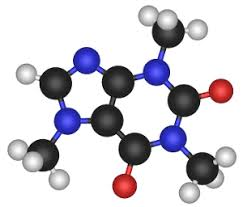The image above represents the molar mass of a substance.

To compute for the molar mass, two essential parameters are needed and these parameters are mass (m) and number of moles (n).

The formula for calculating the molar mass:

M = mn

Where;
M = Molar mass
m = Mass
n = Number of moles

Let’s solve an example;
Find the molar mass of a substance with a mass of 14 kg and number of moles of 28 mol.

This implies that;
m = Mass = 14
n = Number of moles = 28

M = mn
M = 14 ⁄ 28
M = 0.5

Therefore, the molar mass is 0.5 Kg/mol.

Calculating the Mass of a Substance when the Molar Mass and the Number of Moles is Given.

m = nM

Where;
m = Mass
n = Number of moles
M = Molar Mass

Let’s solve an example;
Find the mass of a substance with a molar mass of 30 Kg/mol and number of moles of 15 mol.

This implies that;
n = Number of moles = 15
M = Molar Mass = 30

m = nM
m = 15 x 30
m =  450

Therefore, the mass of a substance is 450 kg.

Calculating the Number of Moles of a Substance when the Molar Mass and the Mass is Given

n = m / M

Where;
n = Number of moles
m = Mass
M = Molar Mass

Let’s solve an example;
Find the number of moles of a substance with a mass of 22 kg and molar mass of 40 Kg/mol.

This implies that;
m = Mass = 22
M = Molar Mass = 40

n = m / M
n = 22 / 40
n = 0.55

Therefore, the number of moles is 0.55 mol.

Nickzom Calculator – The Calculator Encyclopedia is capable of calculating the molar mass.

To get the answer and workings of the molar mass using the Nickzom Calculator – The Calculator Encyclopedia. First, you need to obtain the app.

You can get this app via any of these means:

To get access to the professional version via web, you need to register and subscribe for NGN 1,500 per annum to have utter access to all functionalities.
You can also try the demo version via https://www.nickzom.org/calculator

Once, you have obtained the calculator encyclopedia app, proceed to the Calculator Map, then click on Basic Chemistry under Chemistry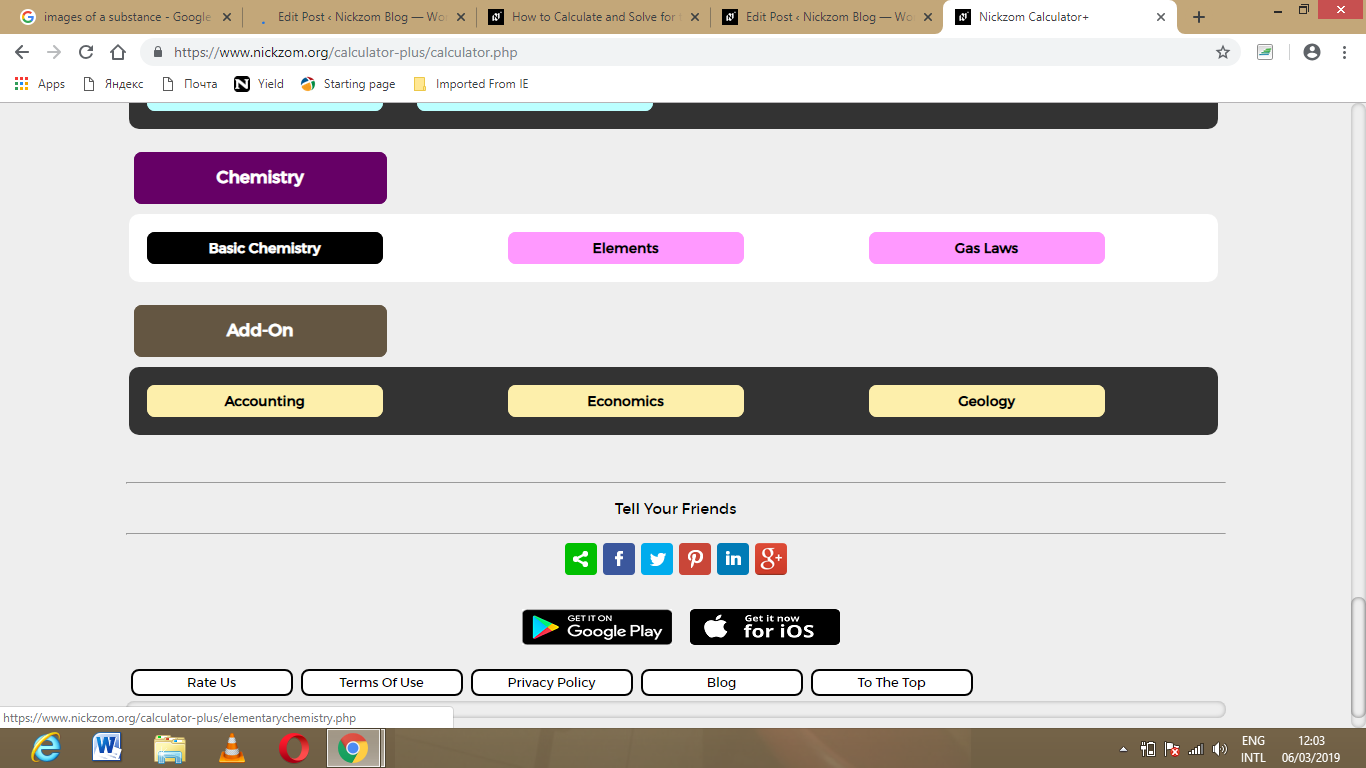Now, Click on Molar Mass under Basic Chemistry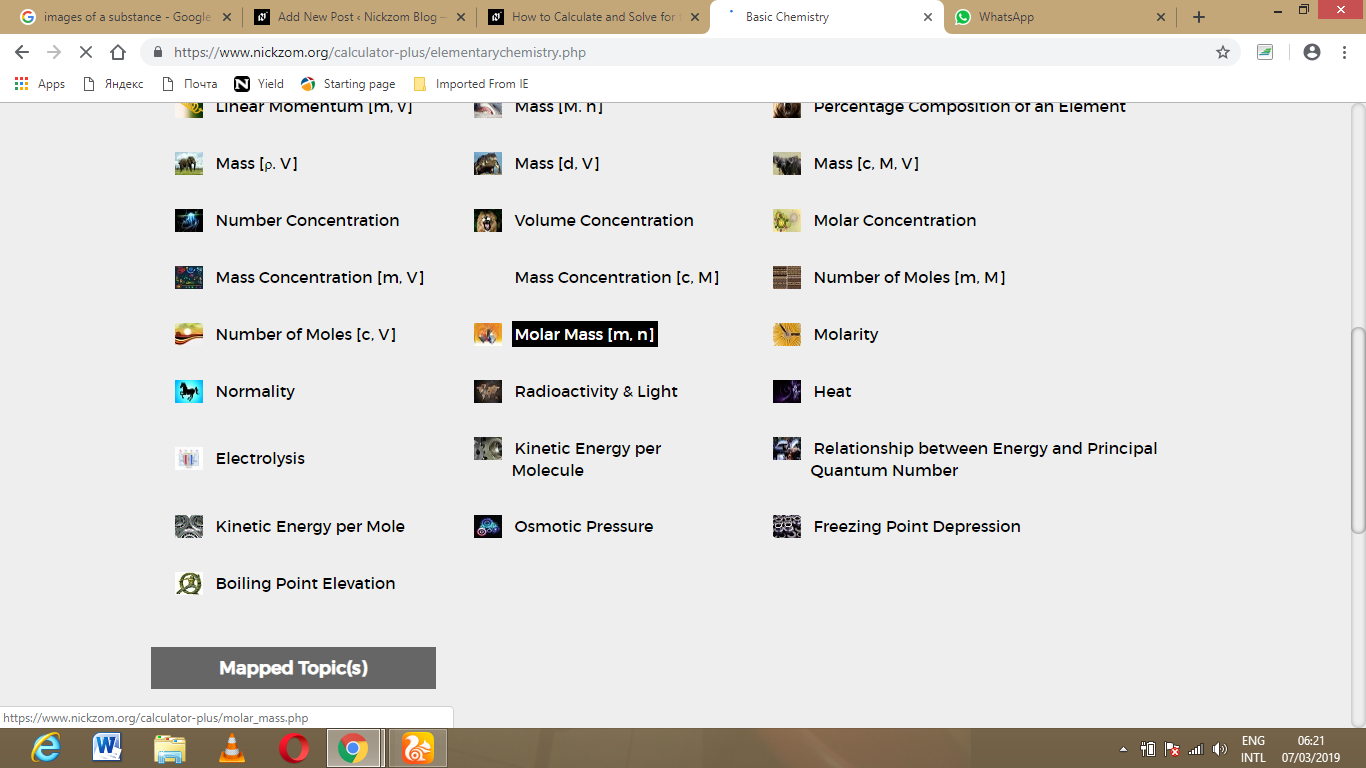The screenshot below displays the page or activity to enter your values, to get the answer for the molar mass according to the respective parameters which are the Mass (m) and Number of moles (n).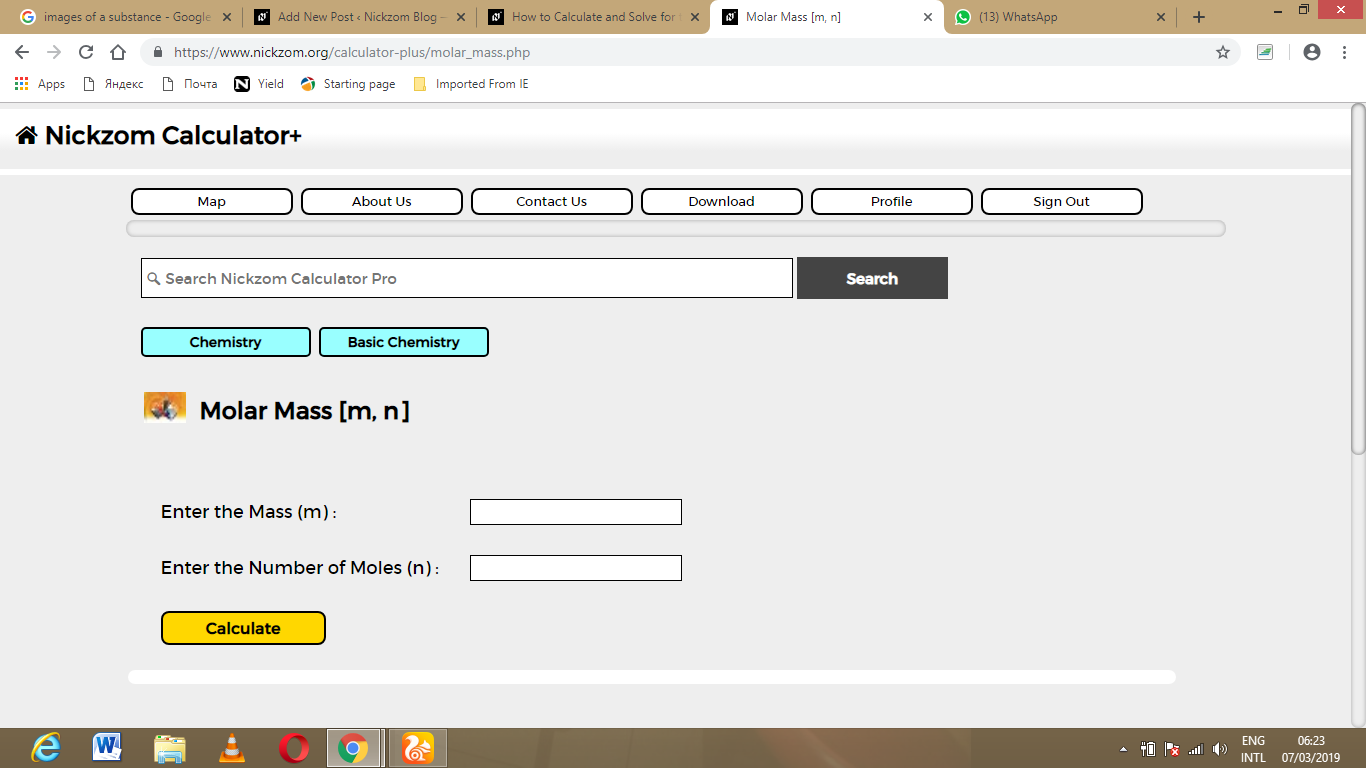Now, enter the values appropriately and accordingly for the parameters as required by the example above where the Mass (m) is 22 kg and Number of moles (n) is 40 kg/mol.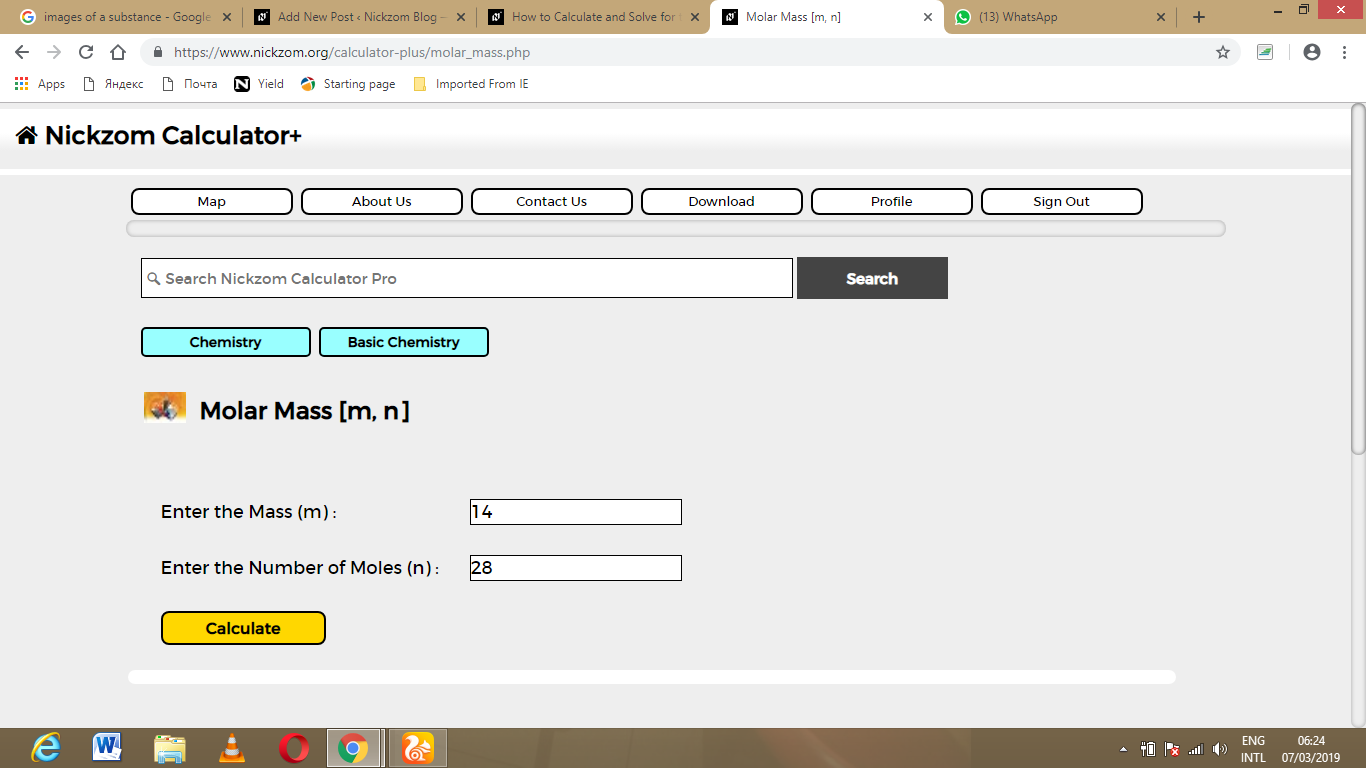Finally, Click on Calculate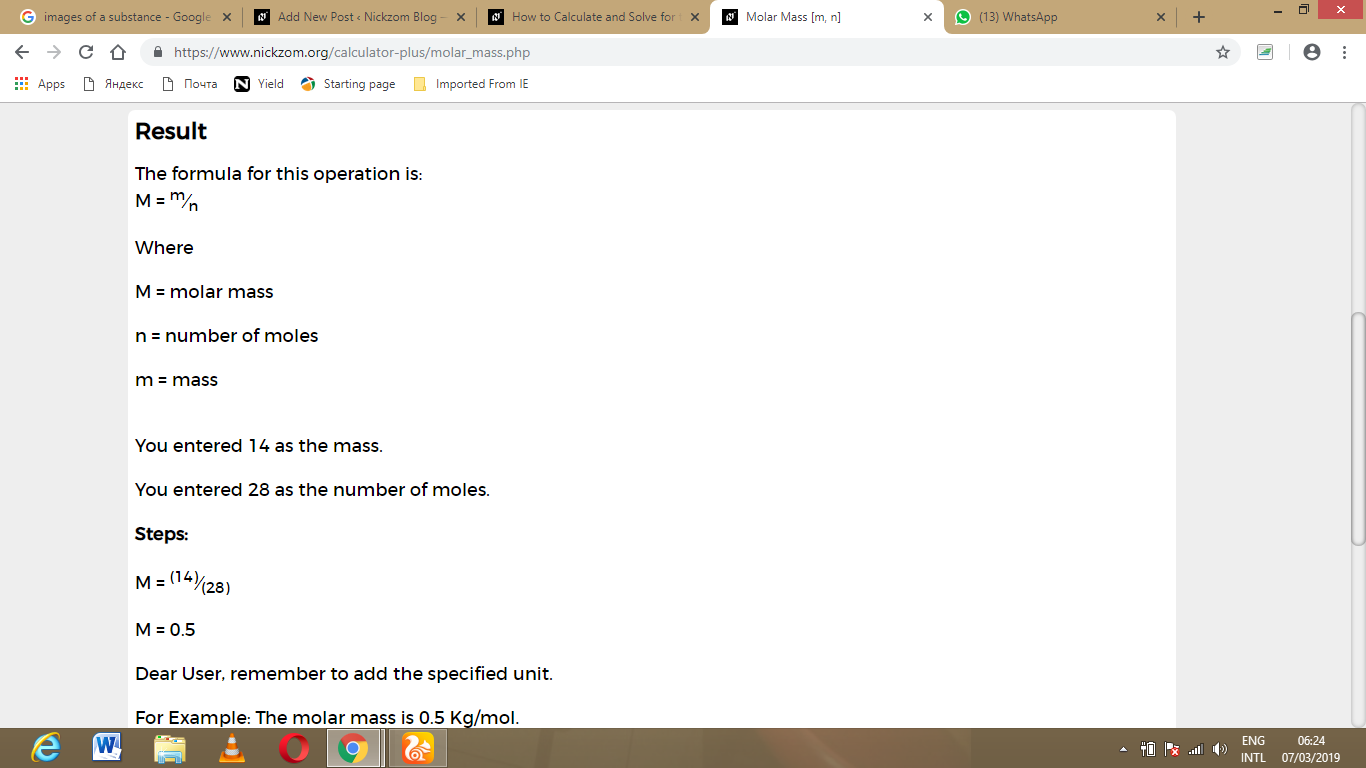As you can see from the screenshot above, Nickzom Calculator – The Calculator Encyclopedia solves for the molar mass and presents the formula, workings and steps too.